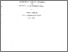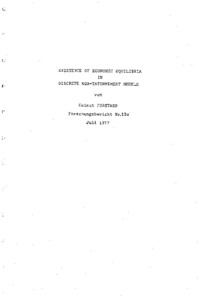# existence of economic equilibria in discrete non-tatonnement models

Forstner, Helmut (July 1977) existence of economic equilibria in discrete non-tatonnement models. Closed Series > Forschungsberichte / Research Memoranda 120Preview
Text
fo120.pdf

Download (2MB) | Preview

## Abstract or Table of Contents

abstract: this paper is intended to describe a monetary pure exchange economy in mathematical terms. three main characteristics of the approach presented are to be mentioned; (i) analysis is carried out in a discrete time framework, (ii) a non-tatonnement process is assumed to represent the dynamics of the economic system, (iii) the problems of the existence and the economic properties of equilibrium states are mainly focused on. by this special choice of methods some results are obtained whichjustify the application of fixed point algorithms for the purpose of approximate calculation of particular non-tatonnement equilibria. investigations start with a general model of a non-tatonnement economy for which the existence of an equiblibria can be proved. in order to get economically interpretable results a special non-tatonnement model is constructed by transforming a set of economic assumptions into mathematical relations. an auxiliary system derived from this special model is then thoroughly analyzed using classical fixed point theorems. from the properties established for the auxiliary model equilibrium theorems concerning the "underlying" economy are obtained.;

Item Type: IHS Series Published 26 Sep 2014 10:34 01 Apr 2016 14:07 https://irihs.ihs.ac.at/id/eprint/120

### Actions (login required)View Item